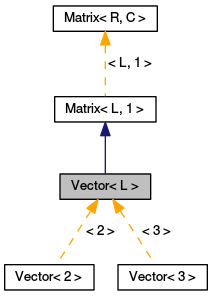MeshKit  1.0
Vector< L > Class Template Reference

Vector is a 1xL Matrix. More...

`#include <Matrix.hpp>`

Inheritance diagram for Vector< L >:[legend]

List of all members.

## Public Member Functions

Vector ()
Vector (double v)
Vector (const double *v)
Vector (const char *s)
Vector (const std::string &s)
Vector (const Matrix< L, 1 > &m)
double & operator[] (unsigned idx)
double operator[] (unsigned idx) const
double & operator() (unsigned idx)
double operator() (unsigned idx) const
Vector< L > & operator= (const Matrix< L, 1 > &m)

## Detailed Description

### template<unsigned L> class MeshKit::Vector< L >

Vector is a 1xL Matrix.

Define a Vector as a 1xL Matrix Add single-index access operators

Definition at line 220 of file Matrix.hpp.

## Constructor & Destructor Documentation

 Vector ( ) ` [inline]`

Definition at line 223 of file Matrix.hpp.

 Vector ( double v ) ` [inline]`

Definition at line 224 of file Matrix.hpp.

 Vector ( const double * v ) ` [inline]`

Definition at line 225 of file Matrix.hpp.

 Vector ( const char * s ) ` [inline]`

Definition at line 226 of file Matrix.hpp.

 Vector ( const std::string & s ) ` [inline]`

Definition at line 227 of file Matrix.hpp.

 Vector ( const Matrix< L, 1 > & m ) ` [inline]`

Definition at line 228 of file Matrix.hpp.

## Member Function Documentation

 double& operator() ( unsigned idx ) ` [inline]`

Definition at line 232 of file Matrix.hpp.

 double operator() ( unsigned idx ) const` [inline]`

Definition at line 233 of file Matrix.hpp.

 Vector& operator= ( const Matrix< L, 1 > & m ) ` [inline]`

Definition at line 235 of file Matrix.hpp.

 double& operator[] ( unsigned idx ) ` [inline]`

Definition at line 230 of file Matrix.hpp.

 double operator[] ( unsigned idx ) const` [inline]`

Definition at line 231 of file Matrix.hpp.

The documentation for this class was generated from the following file: